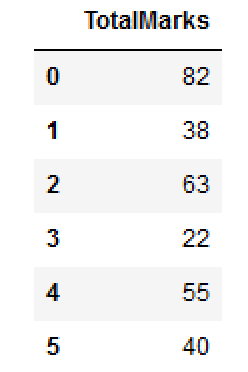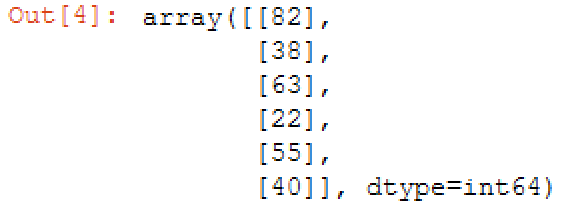Data Science

# Convert Pandas DataFrame to NumPy Array

The seamless interoperability between Pandas and NumPy empowers data analysts and scientists to embark on a transformative journey of data manipulation and analysis. One key facet of this synergy lies in the capability to convert a Pandas DataFrame, a versatile tabular data structure, into a NumPy array, a fundamental and efficient data container in Python.

## Convert pandas dataframe to NumPy array

1. Usng to_numpy()
df.to_numpy()
1. Using to_records()
df.to_records()
1. Using asarray()
np.asarray(df)

Lets craete a DataFrame..

import pandas as pd import numpy as np df = pd.DataFrame() df['TotalMarks'] = [82, 38, 63,22,55,40] dfTotalMarks 0 82 1 38 2 63 3 22 4 55 5 40

The process of converting a Pandas DataFrame to a NumPy array is enabled by the values attribute, which unfolds the DataFrame's contents into a multi-dimensional NumPy array representation. This attribute unlocks a gateway to unlock a treasure trove of data manipulation possibilities, as NumPy arrays offer a streamlined and optimized foundation for numerical computations and mathematical operations.

## Convert pandas dataframe to NumPy array usng to_numpy()

df.to_numpy()
array([, , , , , ], dtype=int64)
Checking Type
type(df.to_numpy())
numpy.ndarray

## NumPy array dtype

In the above output you can see the dtype=int64 . You can also provide dtype=int64 as parameters.

df.to_numpy(dtype ='float32')
array([[82.], [38.], [63.], [22.], [55.], [40.]], dtype=float32)

Once the conversion to a NumPy array is accomplished, data professionals can use the vast ecosystem of NumPy's array-based functionalities. From applying statistical functions and linear algebra operations to employing advanced indexing and slicing techniques, the NumPy array unfolds as a canvas for data exploration and modeling endeavors.

## Convert pandas dataframe to NumPy array usng to_records()

df.to_records()
rec.array([(0, 82), (1, 38), (2, 63), (3, 22), (4, 55), (5, 40)], dtype=[('index', '<i8'), ('TotalMarks', '<i8')])
Checking Type
type(df.to_records())
numpy.recarray

Note that this is a recarray rather than an numpy.ndarray. You could move the result in to regular numpy array by calling its constructor as np.array(df.to_records()) .

np.array(df.to_records())
array([(0, 82), (1, 38), (2, 63), (3, 22), (4, 55), (5, 40)], dtype=(numpy.record, [('index', '<i8'), ('TotalMarks', '<i8')]))
Checking Type
type(np.array(df.to_records()))
numpy.ndarray

The transition from a Pandas DataFrame to a NumPy array is a prudent choice when dealing with large datasets, as NumPy's underlying C implementation grants exceptional computational efficiency and performance. This seamless migration ensures that data-intensive tasks, such as machine learning algorithms or numerical simulations, are executed with precision and speed.

## Convert pandas dataframe to NumPy array usng asarray()

np.asarray(df)array([, , , , , ], dtype=int64)
Checking Type
type(np.asarray(df))
numpy.ndarray

## df.values and df.as_matrix()

You can use df.values and df.as_matrix() to convert dataframe to NumPy array. But these two methods are depreciated. If you visit the v0.24 docs for .values, you will see a big red warning that says:

Warning: We recommend using DataFrame.to_numpy() instead.

### Conclusion

The ability to convert a Pandas DataFrame to a NumPy array represents a foundational step towards unleashing the true potential of data analysis and scientific computing in Python. This harmonious integration of data structures fosters a holistic approach to data-driven solutions, as data professionals can seamlessly traverse between the scope of tabular data manipulation and array-based numerical computations with grace and fluency. By utilizing this interoperability, data analysts and scientists can unlock deeper insights, make data-informed decisions, and drive transformative outcomes with finesse and accuracy.